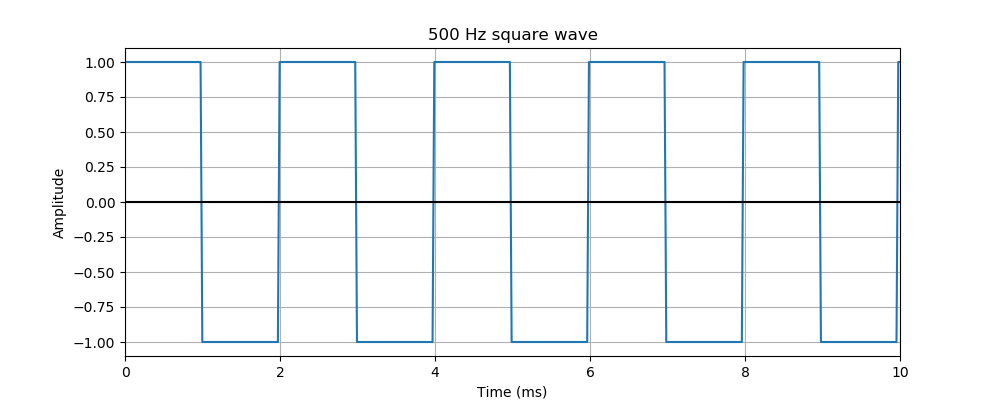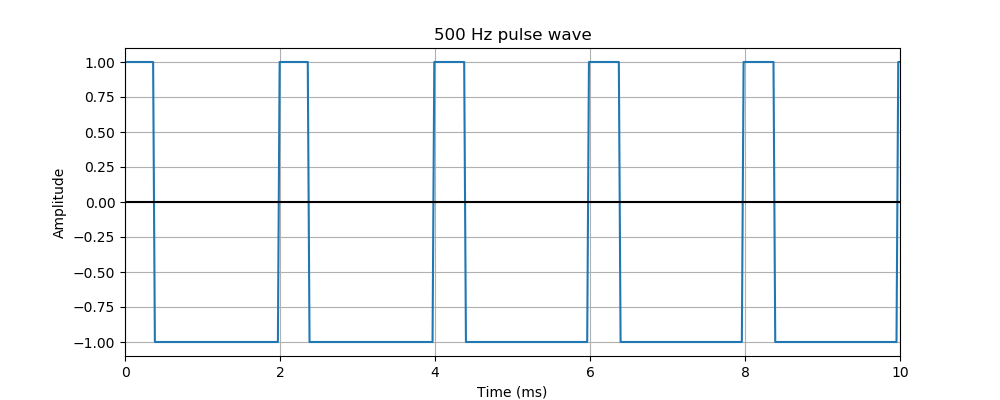# Pysound simple square waves

Martin McBride
2021-10-03

In this example, we will create a simple square wave.

This process is quite similar to the way we create a sine wave, it is worth reading that first.. The way we create a graph of the function, and the way we vary the amplitude and frequency are identical, so we won't repeat them here.

However, square waves have an extra parameter, the ratio, that determines the on/off time of the wave. This creates waves with different shapes, and and similar but different sounds.

## The code

Here is the code:

from pysound.buffer import BufferParams
from pysound.oscillators import sine_wave
from pysound import soundfile
from pysound.graphs import Plotter

params = BufferParams()
out = square_wave(params, frequency=500)
soundfile.save(params, 'square-500.wav', out)


This works in a similar way to the sine wave example, but it uses the square_wave function instead:

out = square_wave(params, frequency=500)


It is stored as square-500.wav in the current folder.

Here is what the sound looks like:This sound is a lot harsher and more electronic sounding than a sine wave.

## Changing the ratio of the square wave

A square wave only has two values, on or off (which are +1.0 and -1.0 in this case). By default, it spends half its time on, and half its time off.

We can change this using the ratio parameter. The ratio specifies the on time as a proportion of the total wave time. A square wave, above, has a ratio of 0.5 (it is on for 50% of the time, therefore off for 50% of the time). The ratio parameter defaults to 0.5, so if you don;t specify it you will get a square wave.

We can change the ratio like this:

out = square_wave(params, frequency=500, ratio=0.2)
soundfile.save(params, 'pulse-500.wav', out)


In this case, the ratio is 0.2 (it is on for 20% of the time, therefore off for 80% of the time). Of course it is also possible to create a wave that is on for longer than it is off (for example a ratio of 0.6 gives a wave that is one for 60% of the time, off for 40%).

A wave with unequal of/off times is sometimes called a pulse wave. Here is what the 0.2 case looks like: